[Next]: Physical modeling and numerical simulation of
 [Up]: Project descriptions
 [Previous]: Simulation of the modulation response of
[Contents]   [Index]

## Dissipative Schrödinger-Poisson systems

Collaborator: M. Baro , H.-Chr. Kaiser , H. Neidhardt , J. Rehberg

Cooperation with: P. Degond, N. Ben Abdallah (Université Paul Sabatier, Toulouse, France), V. Zagrebnov (Université de la Méditerranée (Aix-Marseille II) and Centre de Physique Théorique, France), P. Exner (Academy of Sciences of the Czech Republic, Prague), A. Jüngel (Universität Konstanz), A. Arnold (Westfälische Wilhelms-Universität Münster)

Supported by: DFG: Kopplung von van Roosbroeck- und Schrödinger-Poisson-Systemen mit Ladungsträgeraustausch'' (Coupling between van Roosbroeck and Schrödinger-Poisson systems with carrier exchange)

Description:

The description of semiconductor devices by drift-diffusion models (van Roosbroeck's system) has been widely accepted for a long time. Moreover, there are well-developed software packages for calculating semiconductors based on drift-diffusion models. However, with the developing miniaturization of semiconductor devices less and less atoms are involved in the processes inside many devices with the consequence that quantum effects become more and more important. Hence, one has to improve the classical drift-diffusion description taking quantum effects at least partially into account. It would be more correct to use a complete quantum mechanical description of semiconductors right from the beginning; however, at present it is not possible to handle such a description numerically. Therefore one has to look for a model which uses a drift-diffusion description in regions where this is recommended and a quantum mechanical one in regions where quantum effects are dominating, for instance, in the active zone of a laser. Both descriptions seem to be incompatible. From the physical point of view it would be desirable to have at least a continuity of the current densities at the interface between the two subregions. This leads to the necessity to consider open quantum systems which are, in contrast to closed quantum systems, not yet well developed.

Considering current coupled models one has at first to find open boundary conditions for the Schrödinger operator. In [6, 7], the proposal was made to use for this purpose the Schrödinger operators with non-selfadjoint boundary conditions which in application to a one-dimensional Schrödinger operator H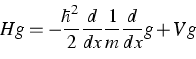(1)
on the interval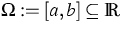, looks like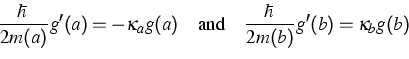(2)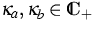. Non-selfadjoint Schrödinger operators of the above type and for higher spatial dimensions have been investigated in . In contrast to self-adjoint boundary conditions, which are used in case of closed Schrödinger-Poisson systems, one has to redefine the carrier and current densities. The best way to find suitable definitions is to close the open system since in this case the definitions are clear. In , the minimal self-adjoint dilation of H was chosen for the Hamiltonian of the covering closed system. The minimal self-adjoint dilation itself was explicitly constructed in  and investigated there in detail. It turned out that the obtained expression of the carrier density is a straightforward generalization of the corresponding expression in the closed case. Indeed, if the carrier density in the closed case is given by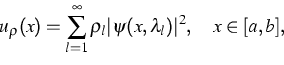(3)
where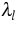,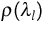and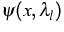denote the eigenvalues, the occupation numbers at the eigenvalueand the eigenfunction corresponding to the eigenvalue, respectively, then in the open case one finds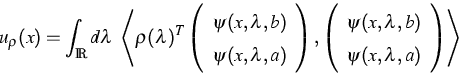(4)
where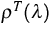is the transposed density matrix and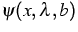,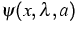are the generalized eigenfunctions of the dilation. Hence one has the following correspondence: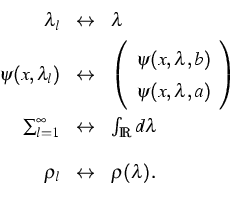(5)
The current density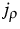, which is always zero in the closed case, is now constant and is, in general, different from zero. Moreover, one gets the representation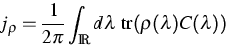(6)
where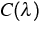is related to the characteristic function of H. Using the redefined notion of carrier density, a dissipative Schrödinger-Poisson system was considered in  and we have shown that this system always has a solution. The electrostatic potential is always bounded by a constant which is determined by the data of the problem. The solution is in general not unique. In  an attempt was made to include generation and recombination effects into the dissipative Schrödinger-Poisson systems.

References:

1. M. BARO, H. NEIDHARDT, Dissipative Schrödinger-type operator as a model for generation and recombination, WIAS Preprint no. 737, 2002, to appear in: J. Math. Phys.
2. H.-CHR. KAISER, H. NEIDHARDT, J. REHBERG, Macroscopic current induced boundary conditions for Schrödinger-type operators, WIAS Preprint no. 650, 2001, to appear in: Integral Equations Operator Theory.
3. M. BARO, H.-CHR. KAISER, H. NEIDHARDT, J. REHBERG, Dissipative Schrödinger-Poisson systems, WIAS Preprint no. 719, 2002.
4. H.-CHR. KAISER, H. NEIDHARDT, J. REHBERG, On 1-dimensional dissipative Schrödinger-type operators, their dilations and eigenfunction expansions, WIAS Preprint no. 664, 2001, to appear in: Math. Nachr.
5., Density and current of a dissipative Schrödinger operator, J. Math. Phys., 43 (2002), pp. 5325-5350.
6. H.-CHR. KAISER, J. REHBERG, About a one-dimensional stationary Schrödinger-Poisson system with Kohn-Sham potential, Z. Angew. Math. Phys., 50 (1999), pp. 423-458.
7., About a stationary Schrödinger-Poisson system with Kohn-Sham potential in a bounded two- or three-dimensional domain, Nonlinear Anal., 41A (2000), pp. 33-72.

 [Next]: Physical modeling and numerical simulation of
 [Up]: Project descriptions
 [Previous]: Simulation of the modulation response of
[Contents]   [Index]

LaTeX typesetting by I. Bremer
5/16/2003